# Integrals of Polynomials

The integral of any polynomial is the sum of the integrals of its terms. A general term of a polynomial can be written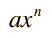and the indefinite integral of that term iswhere a and C are constants. The expression applies for both positive and negative values of n except for the special case of n= -1. In the examples, C is set equal to zero. If definite limits are set for the integration, it is called a definite integral.### Applications

Index

 HyperPhysics*****HyperMath*****Calculus R Nave
Go Back

# Definite Integrals of Polynomials

The definite integral of any polynomial is the sum of the integrals of its terms. A general term of a polynomial can be writtenand the definite integral of that term iswhere b and c are constants, called the limits of the integral. The procedure is basically the same as in the indefinite integral except for the evaluation at the two limits.### Applications

Index

 HyperPhysics*****HyperMath*****Calculus R Nave
Go Back

# Integral of 1/x

As a special case of the integral of a polynomial, the integral of 1/x gives a natural logarithm.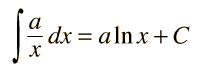where C is a constant of integration.

For the definite integral case: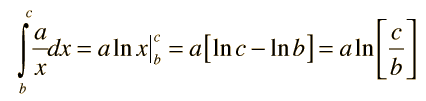### Applications

Index

 HyperPhysics*****HyperMath*****Calculus R Nave
Go Back

# Applications: Integral of 1/x

This integral appears in processes where the rate of change of a variable is proportional to the variable itself. This occurs in radioactive decay: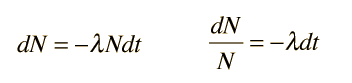Another example is in the calculation of the work done by a gas during an isothermal process. In this case the pressure is inversely proportional to the gas volume V, leading to the work integral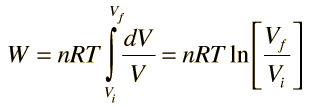Index

 HyperPhysics*****HyperMath*****Calculus R Nave
Go Back

# Polynomial Integrals: Applications

Definite integrals of polynomials show up in many physical applications:

Potential energy of stretched spring:

Moment of Inertia of rod, cylinder, or sphere

Center of mass of rod.

Motion equations for constant acceleration.

Gravitational potential energy at large heights.

Calculating energy stored in a capacitor.

The calculation of voltage difference near a point charge involves a polynomial integral with negative power: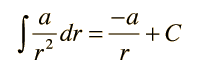An example with a negative exponent is the calculation of work in an adiabatic process.

Index

 HyperPhysics*****HyperMath*****Calculus R Nave
Go Back